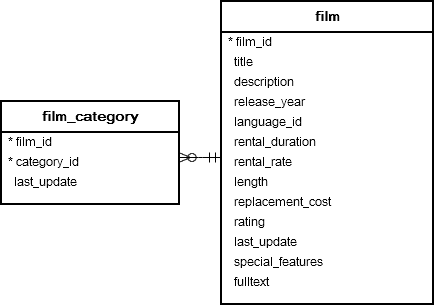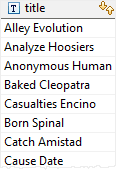# PostgreSQL Tutorial: ANY Operator

August 3, 2023

Summary: in this tutorial, you will learn how to use the PostgreSQL `ANY` operator to compare a scalar value with a set of values returned by a subquery.

## Introduction to PostgreSQL ANY operator

The PostgreSQL `ANY` operator compares a value to a set of values returned by a subquery. The following illustrates the syntax of the `ANY` operator:

``````expresion operator ANY(subquery)
``````

In this syntax:

• The subquery must return exactly one column.
• The `ANY` operator must be preceded by one of the following comparison operator =, <=, >, <, > and <>
• The `ANY` operator returns true if any value of the subquery meets the condition, otherwise, it returns false.

Note that `SOME` is a synonym for `ANY`, meaning that you can substitute `SOME` for `ANY` in any SQL statement.

## PostgreSQL ANY examples

We will use the following `film` and `film_category`tables in the sample database for the demonstration.The following example returns the maximum length of film grouped by film category:

``````SELECT
MAX( length )
FROM
film
INNER JOIN film_category
USING(film_id)
GROUP BY
category_id;
``````

You can use this query as a subquery in the following statement that finds the films whose lengths are greater than or equal to the maximum length of any film category :

``````SELECT title
FROM film
WHERE length >= ANY(
SELECT MAX( length )
FROM film
INNER JOIN film_category USING(film_id)
GROUP BY  category_id );
``````

Here is the result:For each film category, the subquery finds the maximum length. The outer query looks at all these values and determines which film’s lengths are greater than or equal to any film category’s maximum length.

Note that if the subquery does not return any rows, the entire query returns an empty result set.

## ANY vs. IN

The `= ANY` is equivalent to `IN` operator.

The following example gets the film whose category is either `Action` or `Drama`.

``````SELECT
title,
category_id
FROM
film
INNER JOIN film_category
USING(film_id)
WHERE
category_id = ANY(
SELECT
category_id
FROM
category
WHERE
NAME = 'Action'
OR NAME = 'Drama'
);
``````

The result is:The following statement uses the `IN` operator which produces the same result:

``````SELECT
title,
category_id
FROM
film
INNER JOIN film_category
USING(film_id)
WHERE
category_id IN(
SELECT
category_id
FROM
category
WHERE
NAME = 'Action'
OR NAME = 'Drama'
);
``````

Note that the `<> ANY` operator is different from `NOT IN`. The following expression:

``````x <> ANY (a,b,c)
``````

is equivalent to

``````x <> a OR <> b OR x <> c
``````

In this tutorial, you have learned how to use the PostgreSQL `ANY` operator to compare a value to a set of values returned by a subquery.